# Visualize assets¶

ChainerUI provides `/assets` endpoint from v0.8.0 to visualize media assets such image or audio. Basically by using Asset summaries module, functions convert `ndarray` to the specified media type or collect texts, as it turns out to show them on a web browser. Assets page can be seen from `assets` button on result table or result detail.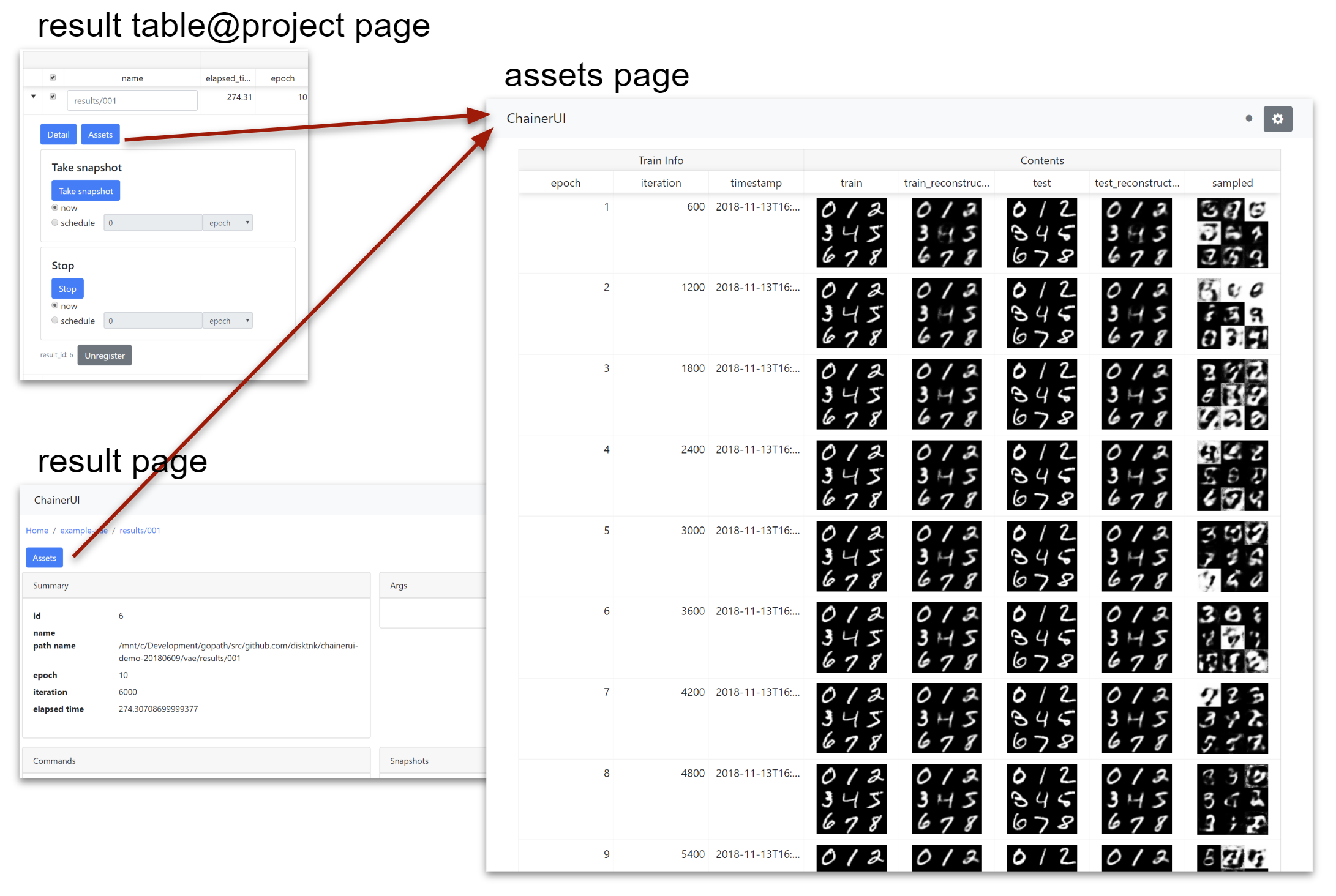Note

`chainerui.summary` module requires output directory path. The path must be same as the directory put `log` file to gather media assets as experimental result. The `log` file is created by LogReport extension or chainerui’s LogReport.

```from chainer import training
from chainerui import summary

out_put = '/path/to/result/'
trainer = training.Trainer(updater, out=out_put)
trainer.extend(training.extensions.LogReport())  # log file will be created at `out_put`
summary.set_out(out_put)  # set output directory as global
```

From next section, example codes are skipped getting assets and set output directory, like blow snippet.

```# get color images and grayscale images for example
import chainer
images, _ = chainer.datasets.get_svhn(withlabel=False)
images_gs, _ = chainer.datasets.get_mnist(withlabel=False, ndim=2)

# make dummy audio data for example
import numpy
audio = numpy.random.uniform(-1, 1, 16000)

from chainerui import summary
summary.set_out('/path/to/result')
```

## Use summary function¶

There are 2 ways to show assets on a web browser. First, use `summary` module function directly. The below example is a simple code to show images.

```summary.image(images[0:5])
summary.image(images[5:10])
summary.audio(audio, 16000)
```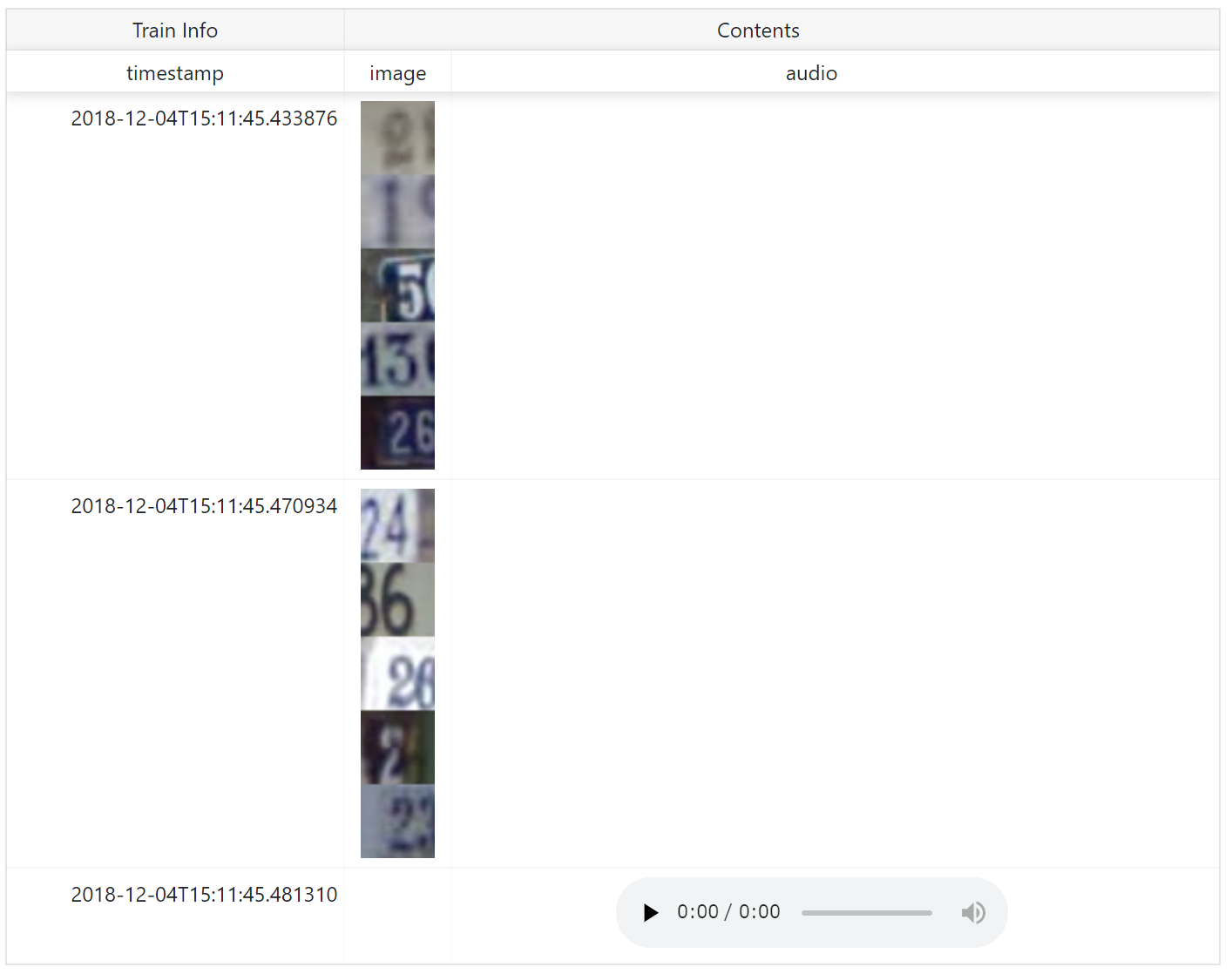`name` is shown as column name. When show assets with additional text information such as epoch number, iteration number, descriptions and so on, add them as `**kwargs`.

```summary.image(images[0:5], name='asset', epoch=1, key='value')
summary.image(images[5:10], name='asset', epoch=2, key='value2')
summary.audio(audio, 16000, name='asset', epoch=3, key='value3')
```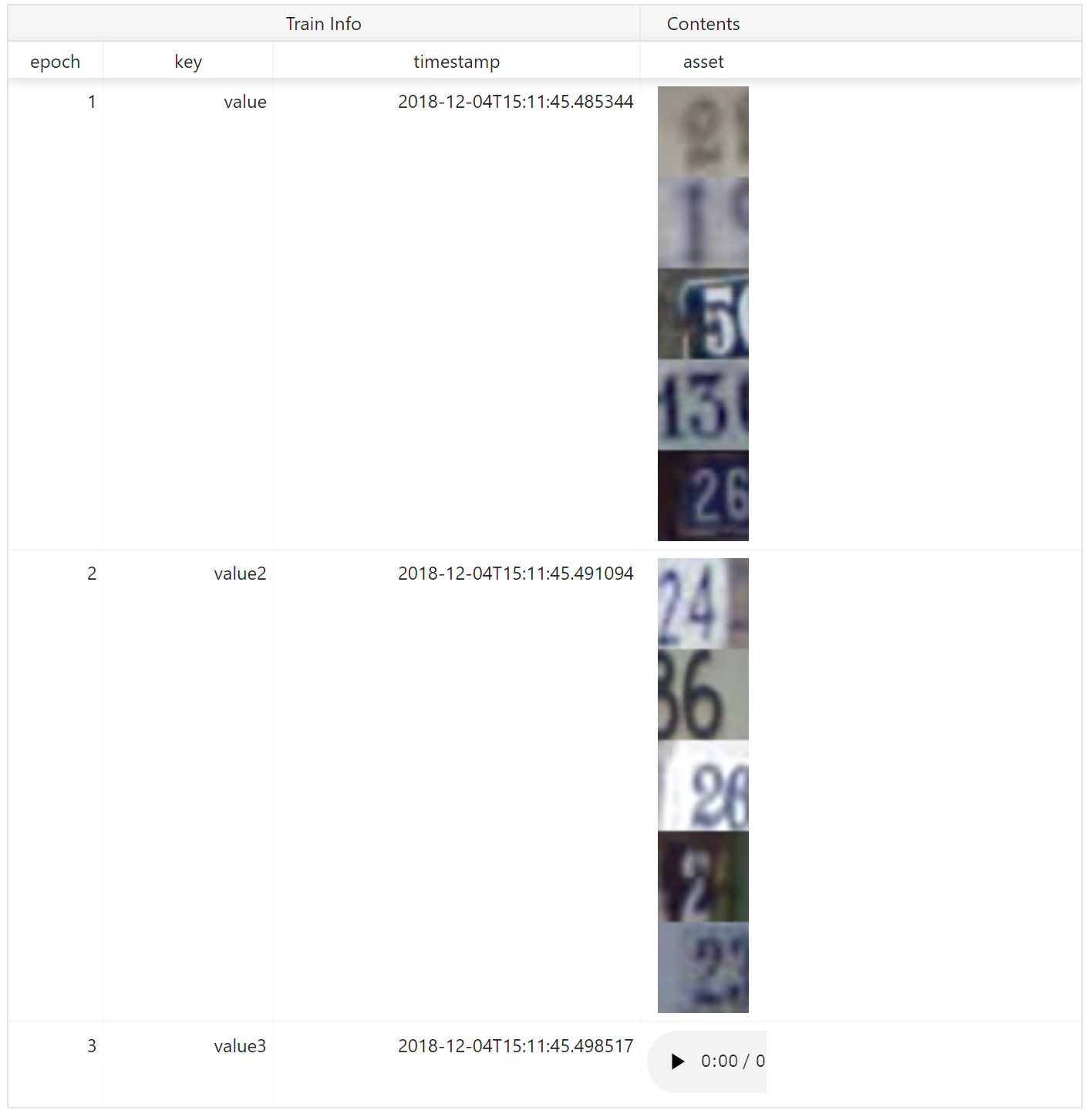## Use `reporter` function¶

Second, to aggregate assets to show them in a same row, use reporter function. Assets called under `with` statement are aggregated.

```with summary.reporter() as r:
r.image(images[0:5])
r.image(images[5:10])
with summary.reporter() as r:
r.image(images[10:15])
r.image(images[15:20])
with summary.reporter() as r:
r.image(images[20:25])
r.image(images[25:30])
```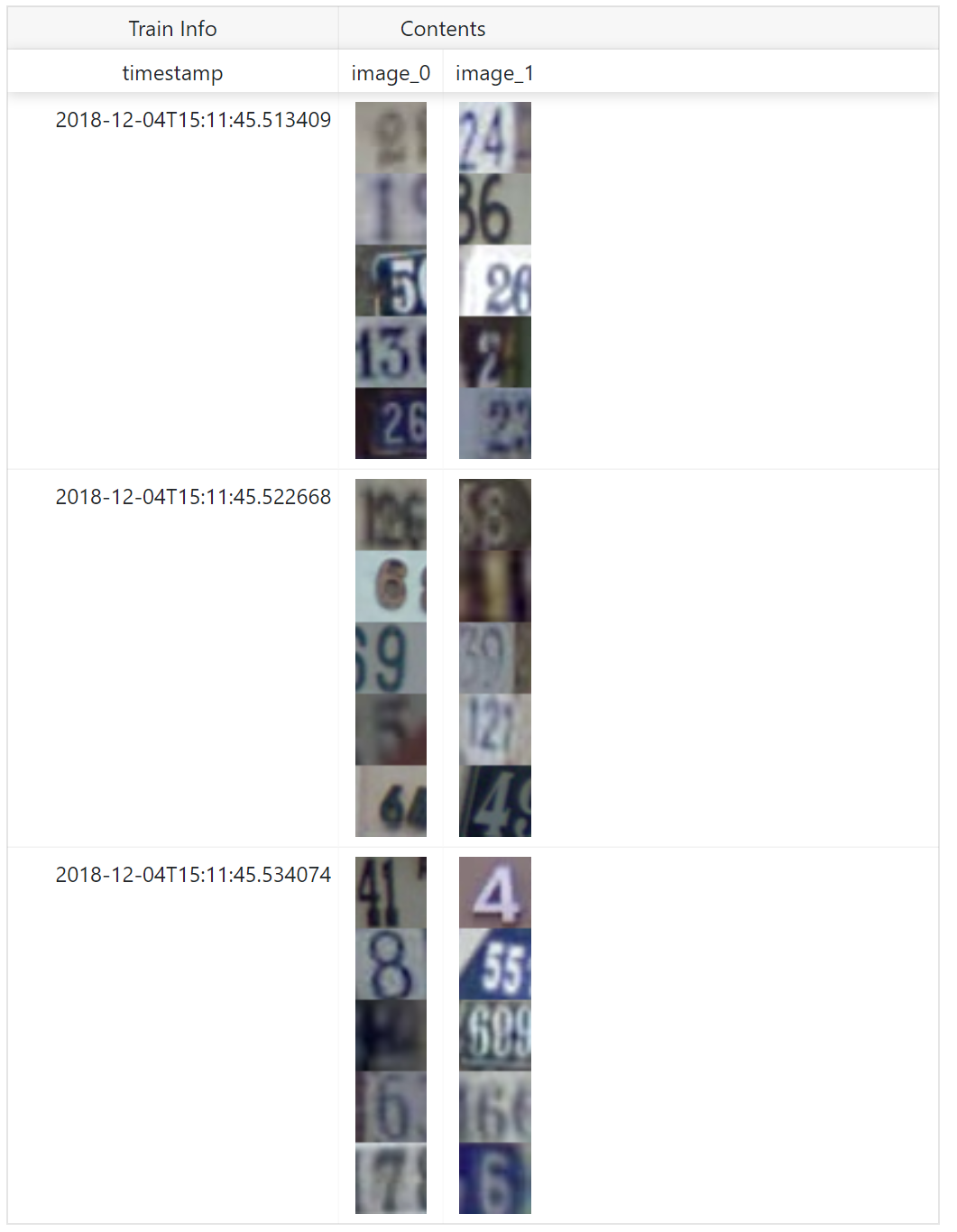`name` is shown as column name. `reporter` also supports `**kwargs` to add other text information.

```with summary.reporter(epoch=1, key='value') as r:
r.image(images[0:5], name='train1')
r.image(images[5:10], name='train2')
with summary.reporter() as r:
r.image(images[10:15], name='train1')
r.image(images[15:20], name='train2')
with summary.reporter() as r:
r.image(images[20:25], name='train1')
r.image(images[25:30], name='train2')
```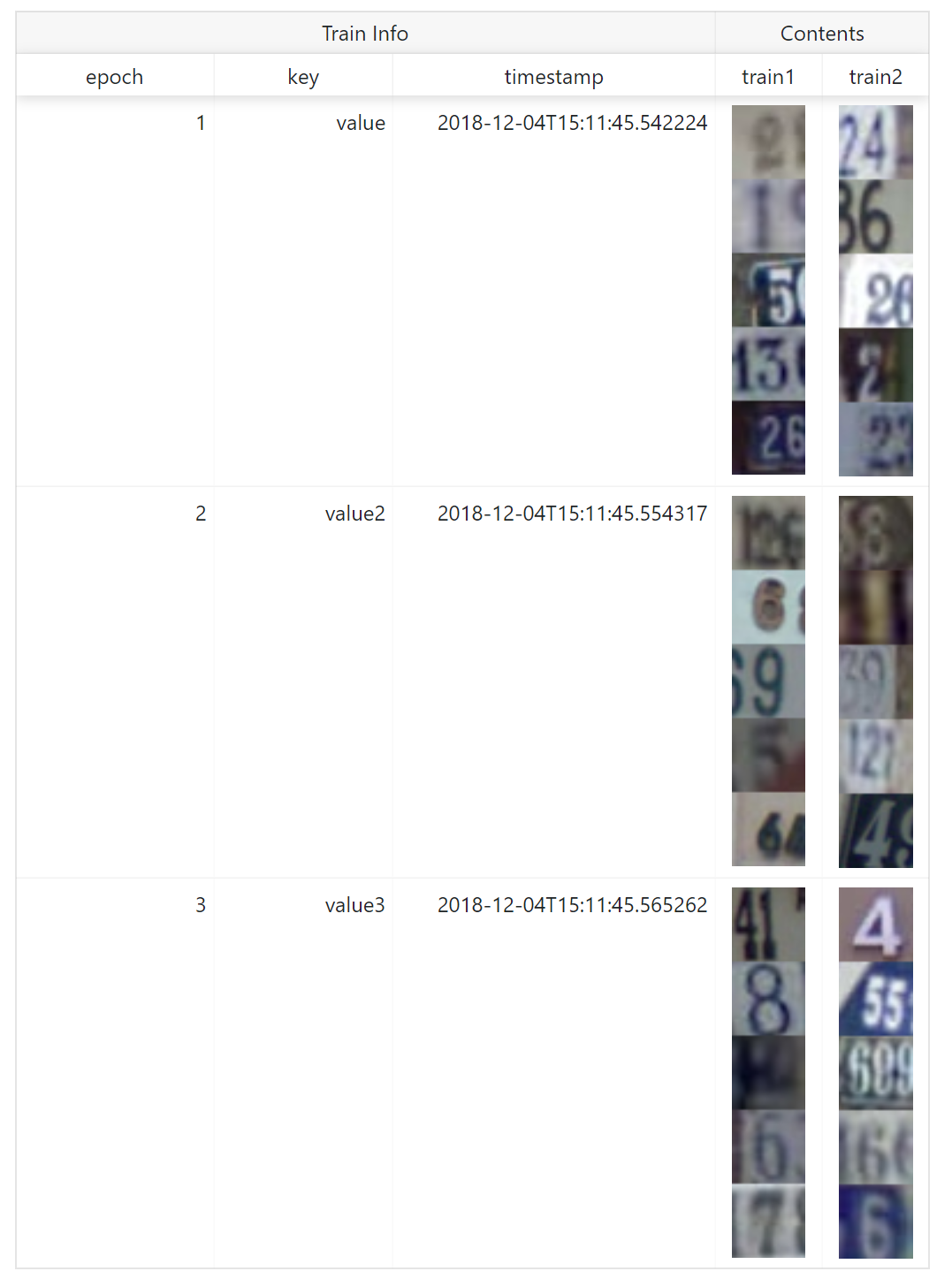## Image¶

Required Pillow to use this function.

Convert `ndarray` to image as PNG format, save, and report to ChainerUI server. `image` function has some options to customize showing.

• Channel position: Dimensions of `ndarray` is considered as batch, channel, height, width on default. If channel is not in 2nd (= `` in 0-origin) dimension, set `ch_axis` option. For example `ndarray` are batch, height, width, channel order, set `ch_axis=-1`.
• Batched or not: Images are considered as bathed array on default. If an array is not batched, set `batched=False`.
• Tiled: Batched array is showed in one line on default. If show tiled them, set `row` option. For example, batch size is 20 and set `row=4`, images are tiled 4x5 on web browser.
• Color space: If images are not `RGB` or `RGBA` color model, set the color mode with `mode` option. ChainerUI support `HSV` color model, set `mode='HSV'`.
• `image(images[0:10])`:• `image(images[0:10], row=1)`:• `image(images[0:10], row=2)`:• `image(images, ch_axis=0, batched=False)`: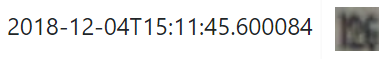• `image(images_gs[0:9])`: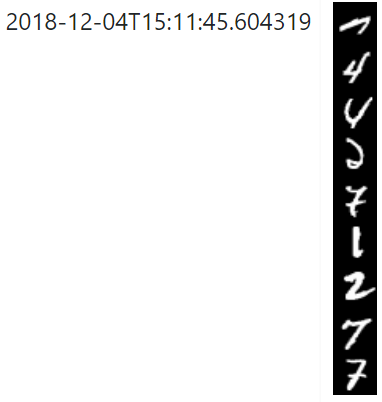• `image(images_gs[0:9], row=1)`:• `image(images_gs[0:9], row=3)`:• `image(images_gs, batched=False)`: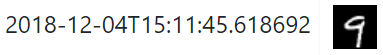## Audio¶

Required Scipy to use this function.

Convert `ndarray` to audio as WAV format, save and report to ChainerUI server. `audio` function requires sample rate.

## Text¶

Simply collect texts, save and report to ChainerUI server.